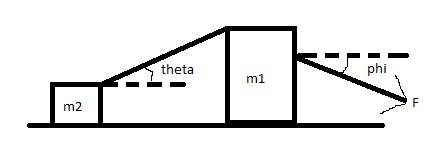# Multi-Mass Force/Acceleration problem

## Homework StatementBox 1 of mass m1 is attached by a thin rope to box 2 of mass m2. Box 1 is pulled by force F. What is the acceleration of box 1 given:
m1= 3kg
m2= 2kg
V(initial)= 4m/s
F= 50N
Friction is present between boxes and floor
μ = 0.2
θ = 20 degrees
∅ = 35 degrees
gravity(g) = 9.8

And what value would F need to be so the velocity is constant at 4.0 m/s

## The Attempt at a Solution

I know that Fnet is going to be the x component of the force F minus the friction between box 1 and the ground and the x component of the tension on box 1 by box 2
Fnet = Fx-(Fμ+Ftx)
By plugging into equations I found
Fx = cos∅*F = 40.9576
Fy = sin∅*F = 28.6788
Fg = m*g = 29.4
N(normal) = Fg+ Fy+Fty=50.0788+Fty
Fμ = N*μ=μ(Fg+Fy+Fty)=0.2(50.0788+Fty)
Fnet =Fx-(Fμ+Ftx)=40.9576-(0.2(50.0788+Fty)+Ftx)
I just can't find Ft
I initially tried that Fty=m2gsinθ and Ftx=m2gcosθ bur this was marked wrong

## Answers and Replies

NihalSh
And what value would F need to be so the velocity is constant at 4.0 m/s

You know that the velocity is constant, what does that tell you???........it tells that net force is 0.....If you correctly balance the forces then you should be able to get the answer, using the above fact. Balance force on both the object (m1 and m2), making a free body diagram should simplify the things.

Last edited:
Yes, I know that much. But that's only for the second half of the problem. I still need the force block 2 pulls on block 1 (Ft, Ftx, Fty) to solve it, and to solve the original problem which is for the acceleration of box 1 given the force of 50 Newtons

nasu
You need to write Newton's second law for each block.
And then solve the coupled equations. You have two unknowns: acceleration and tension in the rope. You can eliminate the tension between the equations and solve for a.

NihalSh
Yes, I know that much. But that's only for the second half of the problem. I still need the force block 2 pulls on block 1 (Ft, Ftx, Fty) to solve it, and to solve the original problem which is for the acceleration of box 1 given the force of 50 Newtons

just like nasu said, and I previously mentioned.

Balance force on both the object (m1 and m2), making a free body diagram should simplify the things.

You will have four equations and four unknowns:
Part A - 2 normal forces, tension and of course the required acceleration
Part B - 2 normal forces, tension and of course the required force F

It should be fairly easy to solve

-----------------------------

You need to write Newton's second law for each block.
And then solve the coupled equations. You have two unknowns: acceleration and tension in the rope. You can eliminate the tension between the equations and solve for a.
don't you think there are four unknowns?

nasu
Yes, you are right if you consider the components as separate equations. Which you should. Sorry for the vague formulation.